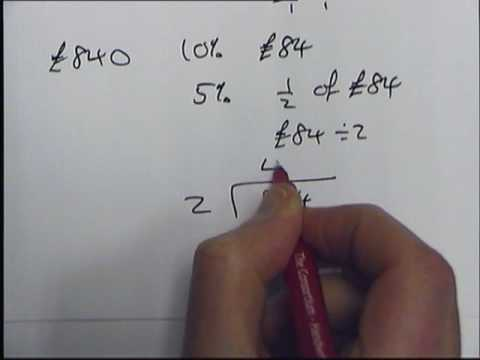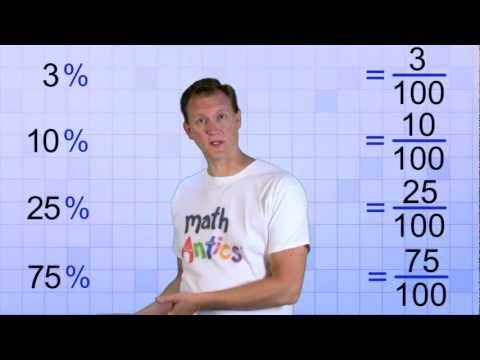## Percentage

Subject: Compulsory Maths

#### Overview

Percent means parts per hundred or out of hundred. The symbol of a percent is %.

#### Percent

Percent means parts per hundred or out of hundred. The symbol of a percent is %.

For example, 25% means 25 per 100 or, $\frac{25}{100}$

Percentage is a fraction written with a denominator 100.

Conversion of fraction or decimal into percent

For the conversion of a fraction or decimal into percent multiply it by 100 and put the symbol % to the product. For example,$\frac{2}{5}$ =$\frac{2}{5}$ × 100 % = 40 %

0.3 = 0.3× 100 % = 30 %

Conversion of percent into fraction or decimal

For the conversion of percent into fraction or decimal, divide it by 100 and remove the % symbol. For example,

20% =$\frac{20}{100}$ =$\frac{1}{5}$

32% =$\frac{32}{100}$ = 0.32

To express a given quantity as the percent of whole quantity

For the expression of the given quantity as the fraction of the whole quantity, then multiply the fraction by 100%. For examples,

20 as the percent of 80 =$\frac{20}{80}$ × 100 = 25%

To find the value of given percent of a quantity

If this case is given, then multiply the quantity by the given percent. Then, convert the percent into a fraction and simplify. For example,

25% of Rs.250 = 25%× 250 =$\frac{25}{100}$× 250 = Rs. 62.5 ans.

To find a quantity whose value of certain percent is given

If this case is given, we can suppose the whole quantity by a variable such as x. Then we can form an equation and by solving the equation we can find the value of x. For example,

If 20% of a sum is Rs. 80. Find the sum

Here, Let the sum be Rs.x

Now, 20% of x = Rs.80

or, $\frac{20}{100}$× x = Rs. 80

or, $\frac{x}{5}$ = Rs.80

or, x =Rs. 80 × 5 = Rs.400

##### Things to remember
• Percentage is a fraction written with a denominator 100.
• For the conversion of a fraction or decimal into percent multiply it by 100 and put the symbol % to the product.
• For the conversion of percent into fraction or decimal, divide it by 100 and remove the % symbol.
• It includes every relationship which established among the people.
• There can be more than one community in a society. Community smaller than society.
• It is a network of social relationships which cannot see or touched.
• common interests and common objectives are not necessary for society.
##### Videos for Percentage##### How to work out percentages without a calculator!!Solution:

Here,
$\frac{3}{20}$ = $\frac{3}{20}$ × 100% = 15%
∴ percentage of absentes = 15%
Now,
the percentage of present students = 100% - 15%
= 85 %

Solution:

Here,
40% = $\frac{40}{100}$ = $\frac{2}{5}$
∴ Fractions of illiterate people = $\frac{2}{5}$
Now,
the fraction of literate people = 1 - $\frac{2}{5}$ = $\frac{3}{5}$

Solution:

Here,
60 as the percentage of 80 = $\frac{60}{80}$ × 100%
75%
So, he obtained 75% marks.

Solution:

Here,
the initial rate of a price = Rs 50 per liter
The new rate of a price = rs 55 per liter
∴ Increment in the rate price = Rs 55 - Rs  50 = rs 5
Now,
the increment percentage = $\frac{Increment \; price}{Initial \; price}$ × 100%
= $\frac{Rs \; 5}{Rs \; 50}$ × 100%
= 10%
So, the rate of a price of petrol is increased by 10%

Solution:

Here,
the total monthly income of the man = Rs 12,000
Expenditure on children's education = 20% of Rs 12,000
= $\frac{20}{100}$ × Rs 12,000
= Rs 2400

Expenditure on food = 25% of Rs 12,000
= $\frac{25}{100}$ × Rs 12,000
= Rs 3,000

Expenditure on rent = 30% of Rs 12,000
= $\frac{30}{100}$ × Rs 12,000
= Rs 3,600

Solution:

Let his monthly income be Rs x.
Here,
His saving percentage = 100% - 65%
= 35%
Now,
or, 35% of x = Rs 2800
or, $\frac{35}{100}$ × x = Rs 2800
or, $\frac{7x}{20}$ = Rs 2800
or, x = $\frac{20 \; × \; Rs 2800}{7}$
or, x = Rs 8000
∴ His monthly income = Rs 8000
Now,
His monthly income = Rs 8000 - Rs 2800
Rs 5200
So, he spends Rs 5200 every month

Solution:

Let, the total number of students in the school be x.
Here,
or, 75% of x = 225
or, $\frac{75}{100}$ × x = 225
or, $\frac{3x}{4}$ = 225
or, x = $\frac{4 × 225}{3}$
or, x = 300
So, the total number of students in the school is 300,
Again,
Number of girls = 300 - 225
So, there are 75 girls in the school.